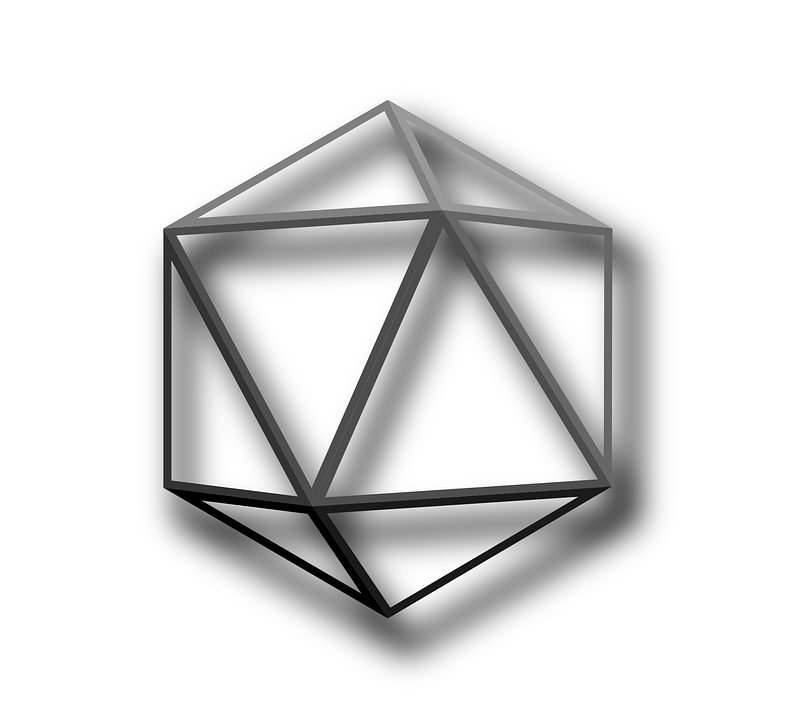# Importance of GeometryGeometry is one of the most significant classical disciplines of Maths. It is important to know about geometry since the world is made up of various shapes and spaces. In geometry, we can describe many shapes; these shapes are especially of two types namely two-dimensional and three-dimensional shapes. Two-dimensional shapes can be drawn on a plane that doesn’t have depth and volume, whereas three-dimensional shapes will have depth or height, which also contain volume.

Some of the examples of two-dimensional shapes are circles, squares, rectangles, triangles, etc., and the examples of three-dimensional shapes are spheres, cones, cylinders, cubes, cuboids, etc., Also, there are many theorems for geometrical shapes such as circle theorem, Pythagoras theorem, etc.,

There are many applications of geometry in a real-life scenario. Also, we can see many shapes- points, lines, curves, angles, two- and three-dimensional shapes, volumes and proportions form the basics of home design as well as geometry.

Geometrical tools like the protractor, ruler, compass. divider, measuring tape, and much more are used in construction work, astronomy, for measurements, drawing, etc. Various art forms are made by joining different geometric shapes together. For a student of an elementary or middle school, geometry is all about different basic shapes including their naming, properties, and formulas related to their areas and volumes.

We will be better prepared to use the relationship between shapes and sizes in our everyday lives when we know how to apply and understand. Geometry will help us in performing that because it gives knowledge on how to deal with measurements and relationships of lines, angles, surfaces, volumes, and solids. For example, if a ball is in the shape of a sphere, if we want to find the weight of the ball, we can use the formula for the volume of sphere.

Geometry is extremely useful in home building or betterment projects. In order to find the floor area of a house, we need to use geometry. Also, in order to replace a piece of furniture, we need to calculate the amount of fabric we want, by calculating the surface area of the furniture.

Those are some of the more basic applications of geometry, but it doesn’t end there. Some more professions use geometry in order to facilitate in their profession, such as in computer science for designing, animation, etc.,

The other application of geometry is calculus, which was strongly influenced by geometry. For example, the introduction of coordinates by and the simultaneous developments of algebra identified a new stage for geometry, since functions and equations could now be represented by geometric figures such as plane curves. This played a significant role in the emergence of infinitesimal calculus. Analytic geometry continues to be a mainstay of precalculus and calculus curriculum. Another important area of application is number theory, for example solving problems in number theory such as finding Pythagorean triples.

There are different branches of geometry namely Euclidean, analytic, projective, differential geometry and non-euclidean geometries. Topology is the latest and most advanced branch of geometry. It focuses on the properties of geometric objects that remain unchanged upon continuous deformation: shrinking, stretching, and folding, but not tearing.